Search by Topic

Resources tagged with Generalising similar to Calcunos:

Filter by: Content type:
Age range:
Challenge level:

There are 141 results

Broad Topics > Using, Applying and Reasoning about Mathematics > Generalising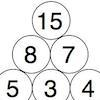Build it Up

Age 7 to 11 Challenge Level:

Can you find all the ways to get 15 at the top of this triangle of numbers? Many opportunities to work in different ways.Button-up Some More

Age 7 to 11 Challenge Level:

How many ways can you find to do up all four buttons on my coat? How about if I had five buttons? Six ...?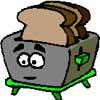Broken Toaster

Age 7 to 11 Short Challenge Level:

Only one side of a two-slice toaster is working. What is the quickest way to toast both sides of three slices of bread?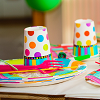Sitting Round the Party Tables

Age 5 to 11 Challenge Level:

Sweets are given out to party-goers in a particular way. Investigate the total number of sweets received by people sitting in different positions.Sums and Differences 1

Age 7 to 11 Challenge Level:

This challenge focuses on finding the sum and difference of pairs of two-digit numbers.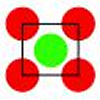Centred Squares

Age 7 to 11 Challenge Level:

This challenge, written for the Young Mathematicians' Award, invites you to explore 'centred squares'.Build it up More

Age 7 to 11 Challenge Level:

This task follows on from Build it Up and takes the ideas into three dimensions!Sums and Differences 2

Age 7 to 11 Challenge Level:

Find the sum and difference between a pair of two-digit numbers. Now find the sum and difference between the sum and difference! What happens?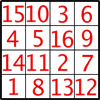Magic Constants

Age 7 to 11 Challenge Level:

In a Magic Square all the rows, columns and diagonals add to the 'Magic Constant'. How would you change the magic constant of this square?Calendar Calculations

Age 7 to 11 Challenge Level:

Try adding together the dates of all the days in one week. Now multiply the first date by 7 and add 21. Can you explain what happens?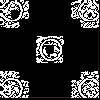Doplication

Age 7 to 11 Challenge Level:

We can arrange dots in a similar way to the 5 on a dice and they usually sit quite well into a rectangular shape. How many altogether in this 3 by 5? What happens for other sizes?Bundles of Cubes

Age 7 to 11 Challenge Level:

Watch this animation. What do you notice? What happens when you try more or fewer cubes in a bundle?Dice Stairs

Age 7 to 11 Challenge Level:

Can you make dice stairs using the rules stated? How do you know you have all the possible stairs?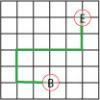Journeys in Numberland

Age 7 to 11 Challenge Level:

Tom and Ben visited Numberland. Use the maps to work out the number of points each of their routes scores.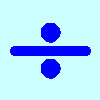Division Rules

Age 7 to 11 Challenge Level:

This challenge encourages you to explore dividing a three-digit number by a single-digit number.Tiling

Age 7 to 11 Challenge Level:

An investigation that gives you the opportunity to make and justify predictions.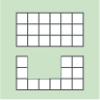Area and Perimeter

Age 7 to 11 Challenge Level:

What can you say about these shapes? This problem challenges you to create shapes with different areas and perimeters.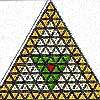The Great Tiling Count

Age 7 to 11 Challenge Level:

Compare the numbers of particular tiles in one or all of these three designs, inspired by the floor tiles of a church in Cambridge.Age 5 to 11 Challenge Level:

Try out this number trick. What happens with different starting numbers? What do you notice?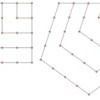Polygonals

Age 7 to 11 Challenge Level:

Polygonal numbers are those that are arranged in shapes as they enlarge. Explore the polygonal numbers drawn here.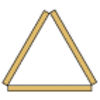Sticky Triangles

Age 7 to 11 Challenge Level:

Can you continue this pattern of triangles and begin to predict how many sticks are used for each new "layer"?Simple Train Journeys

Age 5 to 11 Challenge Level:

How many different journeys could you make if you were going to visit four stations in this network? How about if there were five stations? Can you predict the number of journeys for seven stations?Round the Three Dice

Age 7 to 11 Challenge Level:

What happens when you round these three-digit numbers to the nearest 100?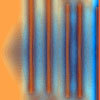Move a Match

Age 7 to 11 Challenge Level:

How can you arrange these 10 matches in four piles so that when you move one match from three of the piles into the fourth, you end up with the same arrangement?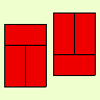Fault-free Rectangles

Age 7 to 11 Challenge Level:

Find out what a "fault-free" rectangle is and try to make some of your own.Round and Round the Circle

Age 7 to 11 Challenge Level:

What happens if you join every second point on this circle? How about every third point? Try with different steps and see if you can predict what will happen.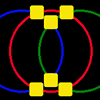Magic Circles

Age 7 to 11 Challenge Level:

Put the numbers 1, 2, 3, 4, 5, 6 into the squares so that the numbers on each circle add up to the same amount. Can you find the rule for giving another set of six numbers?Number Differences

Age 7 to 11 Challenge Level:

Place the numbers from 1 to 9 in the squares below so that the difference between joined squares is odd. How many different ways can you do this?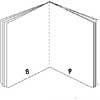Lost Books

Age 7 to 11 Challenge Level:

While we were sorting some papers we found 3 strange sheets which seemed to come from small books but there were page numbers at the foot of each page. Did the pages come from the same book?Cut it Out

Age 7 to 11 Challenge Level:

Can you dissect an equilateral triangle into 6 smaller ones? What number of smaller equilateral triangles is it NOT possible to dissect a larger equilateral triangle into?Oddly

Age 7 to 11 Challenge Level:

Find the sum of all three-digit numbers each of whose digits is odd.Domino Numbers

Age 7 to 11 Challenge Level:

Can you see why 2 by 2 could be 5? Can you predict what 2 by 10 will be?Three Dice

Age 7 to 11 Challenge Level:

Investigate the sum of the numbers on the top and bottom faces of a line of three dice. What do you notice?Crossings

Age 7 to 11 Challenge Level:

In this problem we are looking at sets of parallel sticks that cross each other. What is the least number of crossings you can make? And the greatest?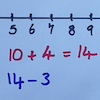Strike it Out

Age 5 to 11 Challenge Level:

Use your addition and subtraction skills, combined with some strategic thinking, to beat your partner at this game.Surprising Split

Age 7 to 11 Challenge Level:

Does this 'trick' for calculating multiples of 11 always work? Why or why not?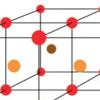Finding 3D Stacks

Age 7 to 11 Challenge Level:

Can you find a way of counting the spheres in these arrangements?Triangle Pin-down

Age 7 to 11 Challenge Level:

Use the interactivity to investigate what kinds of triangles can be drawn on peg boards with different numbers of pegs.Taking Steps

Age 7 to 11 Challenge Level:

In each of the pictures the invitation is for you to: Count what you see. Identify how you think the pattern would continue.Circles, Circles

Age 5 to 11 Challenge Level:

Here are some arrangements of circles. How many circles would I need to make the next size up for each? Can you create your own arrangement and investigate the number of circles it needs?Magic Vs

Age 7 to 11 Challenge Level:

Can you put the numbers 1-5 in the V shape so that both 'arms' have the same total?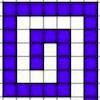Spirals, Spirals

Age 7 to 11 Challenge Level:

Here are two kinds of spirals for you to explore. What do you notice?Odd Squares

Age 7 to 11 Challenge Level:

Think of a number, square it and subtract your starting number. Is the number you’re left with odd or even? How do the images help to explain this?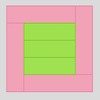Cuisenaire Rods

Age 7 to 11 Challenge Level:

These squares have been made from Cuisenaire rods. Can you describe the pattern? What would the next square look like?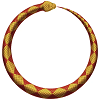Snake Coils

Age 7 to 11 Challenge Level:

This challenge asks you to imagine a snake coiling on itself.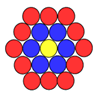Counting Counters

Age 7 to 11 Challenge Level:

Take a counter and surround it by a ring of other counters that MUST touch two others. How many are needed?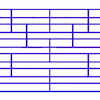Tumbling Down

Age 7 to 11 Challenge Level:

Watch this animation. What do you see? Can you explain why this happens?Truth or Lie

Age 7 to 11 Challenge Level:

Take a look at the video of this trick. Can you perform it yourself? Why is this maths and not magic?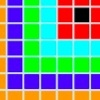Walking the Squares

Age 7 to 11 Challenge Level:

Find a route from the outside to the inside of this square, stepping on as many tiles as possible.Always, Sometimes or Never?

Age 5 to 11 Challenge Level:

Are these statements relating to odd and even numbers always true, sometimes true or never true?